# Math in Focus Grade 2 Chapter 10 Practice 5 Answer Key Rounding Numbers to Estimate

Practice the problems of Math in Focus Grade 2 Workbook Answer Key Chapter 10 Practice 5 Rounding Numbers to Estimate to score better marks in the exam.

## Math in Focus Grade 2 Chapter 10 Practice 5 Answer Key Rounding Numbers to Estimate

Mark each number with an ✗ on the number line.

Round each number to the nearest ten. Circle it on the number line. Then fill in the blanks.

Example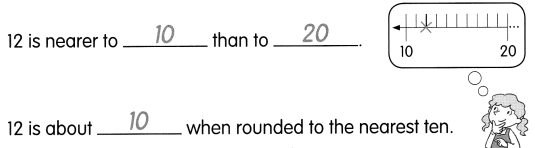Question 1.
48 is nearer to __ than to ____
48 is about ___ when rounded to the nearest ten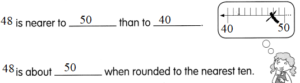48 is nearer to 50 than to 40
48 is about 50 when rounded to the nearest ten

Question 2.
35 is about ________ when rounded to the nearest ten.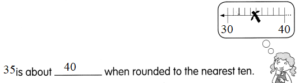35 is about 40 when rounded to the nearest ten.

Question 3.
26 is nearer to _________ than to ___.
26 is about ________ when rounded to the nearest ten.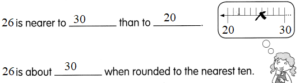26 is nearer to 30 than to 20.
26 is about 30 when rounded to the nearest ten.

Look at the digits in the tens place. Then till in the blanks.

Example

37 is between 30 and 40

Question 4.
86 is between _________ and ____
86 is between 80 and 90

Question 5.
93 is between _________ and ____.
93 is between 90 and 100.

Question 6.
286 is between _______ and ____
286 is between 280 and 290

Question 7.
721 is between ________ and ________
721 is between 720 and 730

Mark each number with an ✗ on the number line. Round each number to the nearest ten. Circle the number on the number line. Then fill in the last column of the table.

Question 8.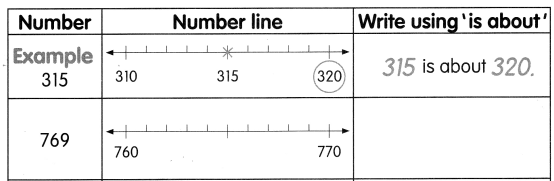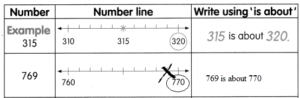Question 9.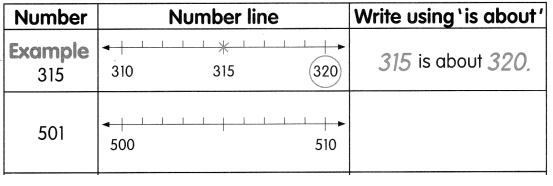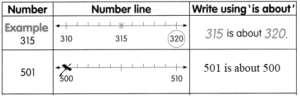Question 10.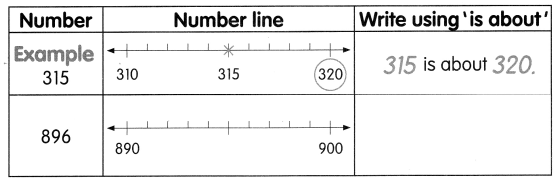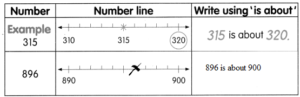Complete the table.

Question 11.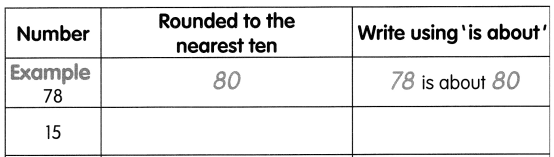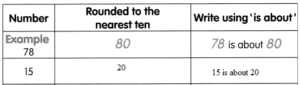Question 12.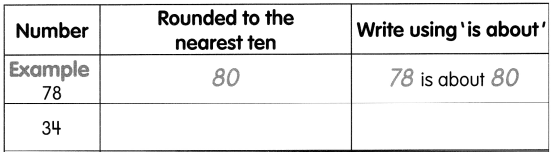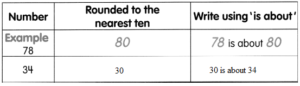Question 13.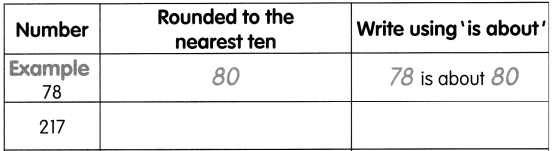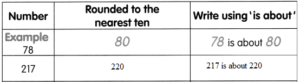Question 14.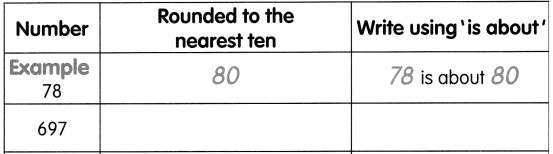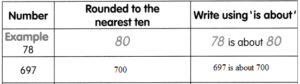Question 15.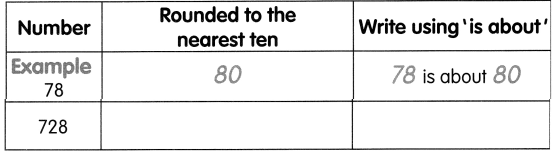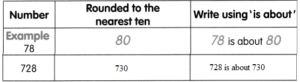The numbers in each problem are rounded to the nearest ten. Find the greatest and least number that is rounded. Use the number line to help you.

Example
Mr. Johnson spent about $80 at a department store.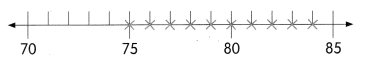The greatest amount he could have spent is$84.

The least amount he could have spent is $75. Question 16. Charles drinks about 790 milliliters of fruit juice in one day.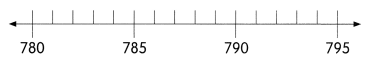The greatest amount he could have had is _________ milliliters. The least amount he could have had is _________ milliliters. Answer: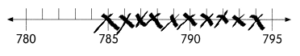The greatest amount he could have had is 794 milliliters. The least amount he could have had is 785 milliliters. Question 17. Shateel ran about 750 meters.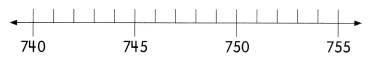The greatest distance she could have run is _________ meters. The least distance she could have run ¡s _________ meters. Answer: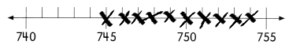The greatest distance she could have run is 754 meters. The least distance she could have run ¡s 745 meters. Question 18. The mass of a bag of potatoes ¡s about 830 grams.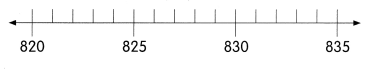The greatest mass of the potatoes could be ___ grams. The least mass of the potatoes could be ___ grams. Answer: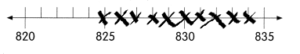The greatest mass of the potatoes could be 834 grams. The least mass of the potatoes could be 825 grams. Find the sum or difference. Then round each number to the nearest ten. Estimate the sum or difference to check that the answers are reasonable. Example 763 + 36 = 799 763 is about 760 36 is about 40 760 + 40 = 800 So, 763 + 36 is about 800 Is the answer reasonable? Explain. Yes, because 800 is close to 799. Answer: Question 19. 238 + 98 = _______ 238 is about _____ 98 is about ____. ___ + ____ = ____ So, 238 + 98 is about ____ Is the answer reasonable? Explain. _____ Answer: 238 + 98 = 336 238 is about 230 98 is about 100. 230 + 100 = 330 So, 238 + 98 is about 330 Explanation : No, As 330 is the nearest tenth value of 336 Question 20. 847 – 95 = _______ 847 is about ____. 95 is about ____. _____ – ____ = _____ So, 846 – 95 = ____ ____ – ____ = ____ Is the answer reasonable? Explain. _______ Answer: 847 – 95 = 752 847 is about 840. 95 is about 90. 840 – 90 = 750 So, 846 – 95 is about 330 847 – 95 = 752 Explanation: Yes, because 750 is close to 752 Question 21. 781 + 49 = _______ Check: _________ + _________ = _____. Is the answer reasonable? Explain. Answer: 781 + 49 = 830 781 is about 780. 49 is about 50. 780 + 50 = 830 Check: 781 + 49 = 830 Explanation: Yes, because 830 is equal to 830 Question 22. 259 – 72 = _______ Check: ________ – ________ = ____. Is the answer reasonable? Explain. Answer: 259 – 72 =187 260 is about 260. 72 is about 70. 260 – 70 = 190 Check: 259 – 72 is about 187 Explanation: Yes, because 190 is close to 187 Question 23. The school principal has$900 to buy items for the school. Round the cost of each item to the nearest ten.
Then estimate the total cost.
a. A set of 4 textbooks costs $96. 96 is _________ when rounded to the nearest ten. Answer: 96 is 100 when rounded to the nearest ten. b. A printer costs$215.
215 is _________ when rounded to the nearest ten.
215 is 210 when rounded to the nearest ten.

c. A camera costs $147. 147 is _________ when rounded to the nearest ten. Answer: 147 is 150 when rounded to the nearest ten. d. A computer costs$385.
385 is _________ when rounded to the nearest ten.
e. The estimated total cost is $___. Answer: We have the rounded values as given below A set of 4 textbooks costs =$100
A printer costs = $210 A camera costs =$150
A computer costs = $380 we get the cost by adding all the above mentioned values. that is$100 + $210 +$150 + $380 =$840